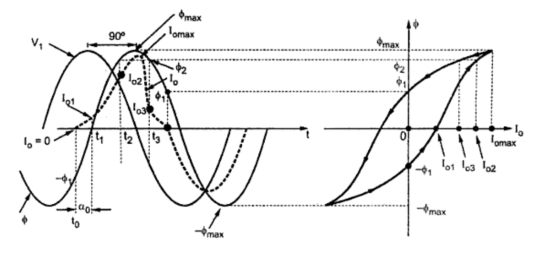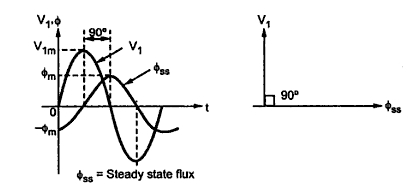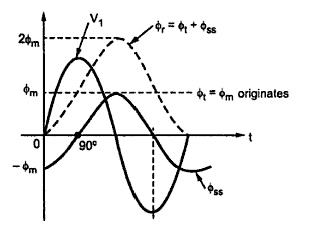### Excitation Phenomenon in Transformers

It is known that though secondary is open, the transformer draws current from supply, when primary is excited by rated voltage. This current is not load current and is basically required to produce core flux. But due to non-linearities of core material such as hysteresis and saturation, the no load current is not sinusoidal in nature. Let us study the effect of hysteresis and saturation on the waveform of no load current which is also called exciting current.
Let V1 be the rated voltage applied to primary. The resistive drop Io R1 is negligible. The applied voltage and induced e.m.f. are sinusoidal in nature. Hence flux in the core also must be sinusoidal. The flux must lag the applied voltage by 90o.
The no load current waveform can be obtained graphically from the hysteresis curve (Φ- Io curve) as shown in the Fig. 1.Fig. 1  Excitation Phenomenon

In the steady state, the flux undergoes a cycle of magnetisation and demagnetisation due to sinusoidal voltage V1 and traces a hysteresis loop as shown in the Fig. 1. Consider the various instants. At t =to, Φ = - Φ1 and no load current Io is zero. At t = t1 , flux is zero and Io = Io1. When Φ = - Φ1 at t = t2, Io =Io1. When Φ = Φ2 at t = t2, Io = Io2. Now Φ2 value occurs twice while Φ increasing and decreasing. But due to hysteresis at t = t3, though Φ = Φ2, Io = Io3. So Io3  Io2 through flux is Φ2. When flux is maximum Φmax, the current Io is also at its maximum Iomax. The current Io again becomes zero at Φ =Φ1.
The negative half will be symmetrical to positive half of Io as hysteresis loop is also symmetrical. It can be seen that the Io waveform is nonsinusoidal and having some peaks. But it is odd symmetrical i.e. current and flux achieve their maxima simultaneously, but current zeros are advanced with respect to flux.
Hence no load current has fundamental and odd harmonics. The strongest is the third harmonic which is about 40% of fundamental. And Io leads flux Φ by a small angle α0. Due to α0, Io has two components Ic and Im as discussed earlier. This phase shift of α0 is caused due to hysteresis nature Φ - Io curve.

Current inrush phenomenon ( Switching transient )
In the steady state operation, both V1 and Φ are sinusoidal and Φ lags V1 by 90° as shown in the Fig.2.Fig.  2

When the primary voltage V1 is switched on to the transformer, the core flux and the exciting current undergo a transient before achieving the steady state. They pass through a transient period. The effect of transient is severe when voltage wave pass through origin.
In the inductive circuit flux can start with zero value. But the steady state value of flux at start is -Φm , as shown in the Fig. 2, at t = 0. Thus during transients a transient flux called off-set flux, Φt = Φm originates such that at t = 0, Φt ss  is zero at the instant of switching. This transient flux Φt then decays according to circuit constants i.e. ratio L/R. This ratio is generally very small for transformers.
Thus during transients, the total flux goes through a maximum value of 2Φm. Such effect is called doubling effect. This is shown in the Fig.3.
Due to the doubling effect, core flux achieves a value of  2Φm due to which transformer draws a large exciting current. This is due to the fact that core goes into deep saturation region of magnetisation. Such a large exiting current can be as large as 100 times the normal exiting current. To withstand electromagnetic forces developed due to large current, the windings of transformer must be strongly braced. This large current drawn during transient is called inrush phenomenon.Fig. 3  Doubling effect

Practically initial core flux can not be zero due to the residual flux Φ'r present, due to retentively property of core. The transient resultant flux goes through Φr = Φ'r + 2Φm and there is heavy inrush current in practice. The effect of transient is even severe in practice.
Such high transient current gradually decreases and finally acquires a steady state. It can last for several seconds. The transient flux Φt and exciting current both are unidirectional during transients. In steady state, exciting current becomes sinusoidal. The Fig. 4 shows the oscillogram of the inrush current.Fig.  4  Waveform of inrush current

Related articles :